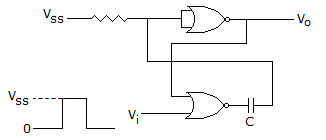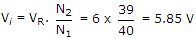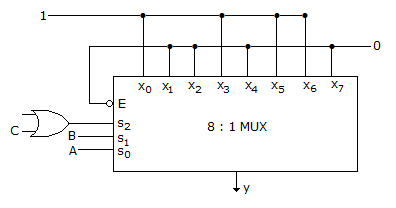# Electronics and Communication Engineering - Digital Electronics

6.

The circuit in the figure is has two CMOS-NOR gates. This circuit functions as aA. flip-flop B. schmitt trigger C. monostable multivibrator D. astable multivibrator

Answer: Option C

Explanation:

It is simple monostable multivibrator.

7.

A 6 bit DAC uses binary weighted resistors. If MSB resistor is 20 k ohm, the value of LSB resistor is

 A. 20 k ohm B. 80 k ohm C. 320 k ohm D. 640 k ohm

Answer: Option D

Explanation:

Resistances are R, 2R, 4R, 8R, 16R and 32R.

LSB resistance = 32R = 32 x 20 = 640 K ohm.

8.

A 6 bit dual slope A/D converter uses a reference of -6v and a 1 MHz clock. It uses a fixed count of 40 (101000). Then, what will be input, if the output register shows 100111 at the end of conversion.

 A. 5.85 V B. 6 V C. 5 V D. 10 V

Answer: Option A

Explanation:I/P voltage and reference voltage arc of opposite polarity.

9.

In the TTL circuit in the figure, S2 to S0 are select lines and X7 to X0 are input lines. S0 and X0 are LSBs. The output Y isA. Indeterminate B. A ⊕ B C. A ⊕ B D. C . A ⊕ B + C . (A ⊕ B)

Answer: Option B

Explanation:

The MUX is made up of TTL circuit. For TTL circuit open terminal is taken high, since S2 select line is connected to OR gate whose one terminal connected to C and the other is open (high) so OR gate output is S2 = 1 + C = 1.

```S2 = 1 S1(B) S0(A)  Y
1       0     0     0
1       0     1     1
1       1     0     1
1       1     1     0```

Y = S0⊕S1 => A⊕B.

10.

The number of accumulators in 6800 are

 A. 2 B. 3 C. 1 D. 4

Answer: Option A

Explanation:

No answer description available for this question. Let us discuss.

#### Current Affairs 2021

Interview Questions and Answers